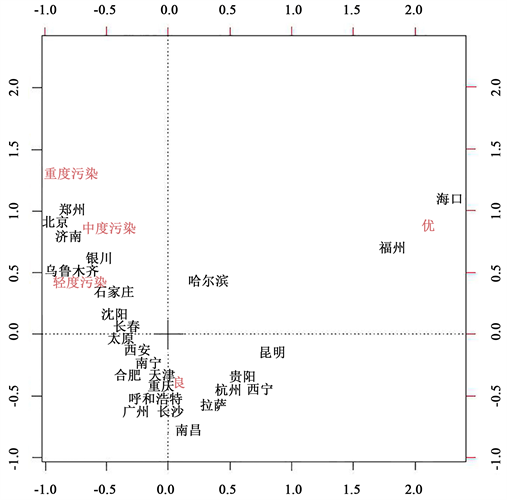# 对31个主要城市空气污染的研究 The Study on Air Pollution in 31 Main Cities

DOI: 10.12677/SA.2019.83064, PDF, HTML, XML, 下载: 402  浏览: 676

Abstract: Based on correspondence analysis and canonical correlation analysis, the relationship between air pollution and cities in 31 main cities is analyzed in this paper. Through the corresponding analysis and research, we can simply classify different cities and analyze the different factors of air quality due to factors such as location conditions and industrial layout. Then, through the typical correlation analysis, it is studied which kinds of pollutants will affect PM2.5 and PM10 in the air quality index, and reasonable improvement suggestions are proposed for these pollutants.

1. 研究背景

2. 数据收集与整理Table 1. Air quality data for 2015 (top five cities)Table 2. Air pollutant concentrations in major cities by month (top five cities)Table 3. Emissions of major pollutants in exhaust gas of major cities in 2015 (top five cities)

3. 基于对应分析与典型相关分析的数据处理与解读

3.1. 对应分析原理

$X={\left({x}_{ij}\right)}_{n×p}$ (3.1.1)

${x}_{i.}=\underset{j=1}{\overset{p}{\sum }}{x}_{ij}$${x}_{.j}=\underset{i=1}{\overset{n}{\sum }}{x}_{ij}$${x}_{..}=\underset{i=1}{\overset{n}{\sum }}\underset{j=1}{\overset{p}{\sum }}{x}_{ij}$ (3.1.2)

1) 由数据矩阵X计算规格化的概率矩阵 $P={\left({p}_{ij}\right)}_{n×p}$

2) 计算过渡矩阵

$Z=\left({z}_{ij}\right)={\left[\left({p}_{ij}-{p}_{i.}{p}_{.j}\right)/\sqrt{{p}_{i.}{p}_{.j}}\right]}_{n×p}={\left(\frac{{x}_{ij}-{x}_{i.}{x}_{.j}/{x}_{..}}{\sqrt{{x}_{i.}{x}_{.j}}}\right)}_{n×p}$ (3.1.3)

3) 进行因子分析

a) R型因子分析：计算协方差矩阵 $A={Z}^{\text{T}}Z$ 的特征值 ${\lambda }_{1},{\lambda }_{2},\cdots ,{\lambda }_{p}$，按照累积百分比 $\underset{i=1}{\overset{m}{\sum }}{\lambda }_{i}/\underset{i=1}{\overset{p}{\sum }}{\lambda }_{i}\ge 85%$，取前m个特征值 ${\lambda }_{1},{\lambda }_{2},\cdots ,{\lambda }_{m}$，并计算对应的单位特征向量 ${u}_{1},{u}_{2},\cdots ,{u}_{m}$，得到因子荷载矩阵：

$F=\left[\begin{array}{cccc}{u}_{11}\sqrt{{\lambda }_{1}}& {u}_{12}\sqrt{{\lambda }_{2}}& \cdots & {u}_{1m}\sqrt{{\lambda }_{m}}\\ {u}_{21}\sqrt{{\lambda }_{1}}& {u}_{22}\sqrt{{\lambda }_{2}}& \cdots & {u}_{2m}\sqrt{{\lambda }_{m}}\\ ⋮& ⋮& & ⋮\\ {u}_{p1}\sqrt{{\lambda }_{1}}& {u}_{p2}\sqrt{{\lambda }_{2}}& \cdots & {u}_{pm}\sqrt{{\lambda }_{m}}\end{array}\right]$ (3.1.4)

b) Q型因子分析：由上述求得的特征值，计算 $B=Z{Z}^{\text{T}}$ 所对应的单位特征向量 ${v}_{i}=\frac{1}{\sqrt{{\lambda }_{i}}}Z{u}_{i}$，得到因子荷载矩阵

$G=\left[\begin{array}{cccc}{v}_{11}\sqrt{{\lambda }_{1}}& {v}_{12}\sqrt{{\lambda }_{2}}& \cdots & {v}_{1m}\sqrt{{\lambda }_{m}}\\ {v}_{21}\sqrt{{\lambda }_{1}}& {v}_{22}\sqrt{{\lambda }_{2}}& \cdots & {v}_{2m}\sqrt{{\lambda }_{m}}\\ ⋮& ⋮& & ⋮\\ {v}_{p1}\sqrt{{\lambda }_{1}}& {v}_{p2}\sqrt{{\lambda }_{2}}& \cdots & {v}_{pm}\sqrt{{\lambda }_{m}}\end{array}\right]$ (3.1.5)

c) 在同一坐标轴上作变量点图与样品点图：分析变量点之间的关系；分析样品点之间的关系；同时综合分析变量点和样品点之间的关系。

3.2. 典型相关分析原理

$\Sigma =Cov\left(x,y\right)=\left[\begin{array}{cc}Var\left(x\right)& Cov\left(x,y\right)\\ Cov\left(y,x\right)& Var\left(y\right)\end{array}\right]=\left[\begin{array}{cc}{\Sigma }_{11}& {\Sigma }_{12}\\ {\Sigma }_{21}& {\Sigma }_{22}\end{array}\right]$ (3.2.1)

$\left\{\begin{array}{l}u={a}_{1}{x}_{1}+{a}_{2}{x}_{2}+\cdots +{a}_{p}{x}_{p}={a}^{\text{T}}x\\ v={b}_{1}{y}_{1}+{b}_{2}{y}_{2}+\cdots +{b}_{q}{y}_{q}={b}^{\text{T}}y\end{array}$ (3.2.2)

u和v的方差和协方差分别为：

$\begin{array}{l}Var\left(u\right)=Var\left({a}^{\text{T}}x\right)={a}^{\text{T}}Var\left(x\right)a={a}^{\text{T}}{\Sigma }_{11}a\\ Var\left(v\right)=Var\left({b}^{\text{T}}y\right)={b}^{\text{T}}Var\left(y\right)b={b}^{\text{T}}{\Sigma }_{22}b\\ Cov\left(u,v\right)=Cov\left({a}^{\text{T}}x,{b}^{\text{T}}y\right)={a}^{\text{T}}Cov\left(x,y\right)b={a}^{\text{T}}{\Sigma }_{12}b\end{array}$ (3.2.3)

$\rho =Corr\left(u,v\right)=Corr\left({a}^{\text{T}}x,{b}^{\text{T}}y\right)=\frac{{a}^{\text{T}}{\Sigma }_{12}b}{\sqrt{\left({a}^{\text{T}}{\Sigma }_{11}a\right)\left({b}^{\text{T}}{\Sigma }_{22}b\right)}}$ (3.2.4)

$\left\{\begin{array}{l}Var\left(u\right)=Var\left({a}^{\text{T}}x\right)={a}^{\text{T}}Var\left(x\right)a={a}^{\text{T}}{\Sigma }_{11}a=1\\ Var\left(v\right)=Var\left({b}^{\text{T}}y\right)={b}^{\text{T}}Var\left(y\right)b={b}^{\text{T}}{\Sigma }_{22}b=1\end{array}$ (3.2.5)

$G={a}^{\text{T}}{\Sigma }_{12}b-\frac{\lambda }{2}\left({a}^{\text{T}}{\Sigma }_{11}a-1\right)-\frac{\mu }{2}\left({b}^{\text{T}}{\Sigma }_{22}b-1\right)$ (3.2.6)

$\left\{\begin{array}{l}{a}^{\text{T}}{\Sigma }_{12}b=\lambda \cdot {a}^{\text{T}}{\Sigma }_{11}a=\lambda \\ {b}^{\text{T}}{\Sigma }_{21}a=\mu \cdot {b}^{\text{T}}{\Sigma }_{22}a=\mu \end{array}$ (3.2.7)

${\left({b}^{\text{T}}{\Sigma }_{21}a\right)}^{\text{T}}={a}^{\text{T}}{\Sigma }_{21}b=\rho$，所以 $\lambda =\mu =\rho$，即 $\lambda$ 恰好就是u和v的相关系数。

$\left\{\begin{array}{l}Aa={\lambda }^{2}a\\ Bb={\lambda }^{2}b\end{array}$ (3.2.8)

${\lambda }^{2}$ 既是A的特征根又是B的特征根，a和b是其相应的特征向量。

$\left\{\begin{array}{l}{u}_{i}={a}_{i1}{x}_{1}+{a}_{i2}{x}_{2}+\cdots +{a}_{ip}{x}_{p}={a}_{i}^{\text{T}}x\\ {v}_{i}={b}_{i1}{y}_{1}+{b}_{i2}{y}_{2}+\cdots +{b}_{ip}{y}_{p}={b}_{i}^{\text{T}}y\end{array}$ (3.2.9)

3.3. 基于对应分析研究城市与空气质量之间的关系Figure 1. Correspondence analysis map of air pollution in major cities of China

3.4. 基于典型相关分析研究主要城市废气排放对空气质量指标的影响

1) 在对城市废气排放与空气中CO、SO2和NO2等指标进行典型相关分析，相关性如下表4所示，发现两者并不存在直接的相关关系。这与一般的认知并不相符，经分析后认为，由于选取全国31个主要城市，存在有不同的区位条件，因此存在大气扩散条件不同的因素，而在空气质量中，这也是很重要的影响因素  。所以判断，空气质量受不同城市的风力等大气扩散条件影响。Table 4. Canonical correlation analysis

2) 鉴于城市区位扩散条件的影响，于是对空气中CO、SO2、NO2和PM2.5、PM10等空气指标进行典型性相关分析，由于这些数据是直接决定空气质量的指标，所以不存在受不可控因素干扰检验的可能。经检验前两列典型相关变量相关系数显著，但根据相关性结果看，第一组相关性较好，结果如下表5~7所示。Table 5. Canonical correlation analysisTable 6. Canonical correlation analysis for UiTable 7. Canonical correlation analysis for Vi

$\begin{array}{l}{U}_{1}=0.009718337{X}_{1}+0.174149964{X}_{2}\\ {V}_{1}=0.05071641{Y}_{1}+0.09547422{Y}_{2}+0.07978821{Y}_{3}+0.01023794{Y}_{4}\end{array}$

$\begin{array}{l}{U}_{1}=0.357534{X}_{1}-0.3124048{X}_{2}\\ {V}_{1}=0.001070345{Y}_{1}-0.188519541{Y}_{2}+0.20400649{Y}_{3}-0.012659033{Y}_{4}\end{array}$

4. 结论

  王科富. 空气污染与肺部健康[D]: [博士学位论文]. 北京: 北京协和医学院, 2016: 6.  王必哲, 吕王勇, 余文瀚, 谢佳益. 基于对应分析法对城市和污染关系的研究[J]. 资源与环境, 2017(4): 113-114.  贺祥. PM2.5浓度变化驱动机制研究——以长江三角洲为例[D]: [博士学位论文]. 南京: 南京师范大学, 2017: 5.  Mitra, R.L.S. (2014) Corruption, Pollution, and the Kuznets Environment Curve. Journal of Environmental Economics and Man-agement, 40, 137-150. https://doi.org/10.1006/jeem.1999.1107  李钢, 蒋文静, 徐洁. 应用灰理论对郑州空气主要污染物分析与预测[J]. 河南城建学院学报, 2013, 22(4): 41-59.  徐承凤. 长沙市产业结构与空气污染关系的实证研究[J]. 经贸实践, 2018(5): 36-37.  张晓娟. 郑州市空气污染指数与地面气象要素相关条件分析[J]. 气象科学, 2015(18): 200.